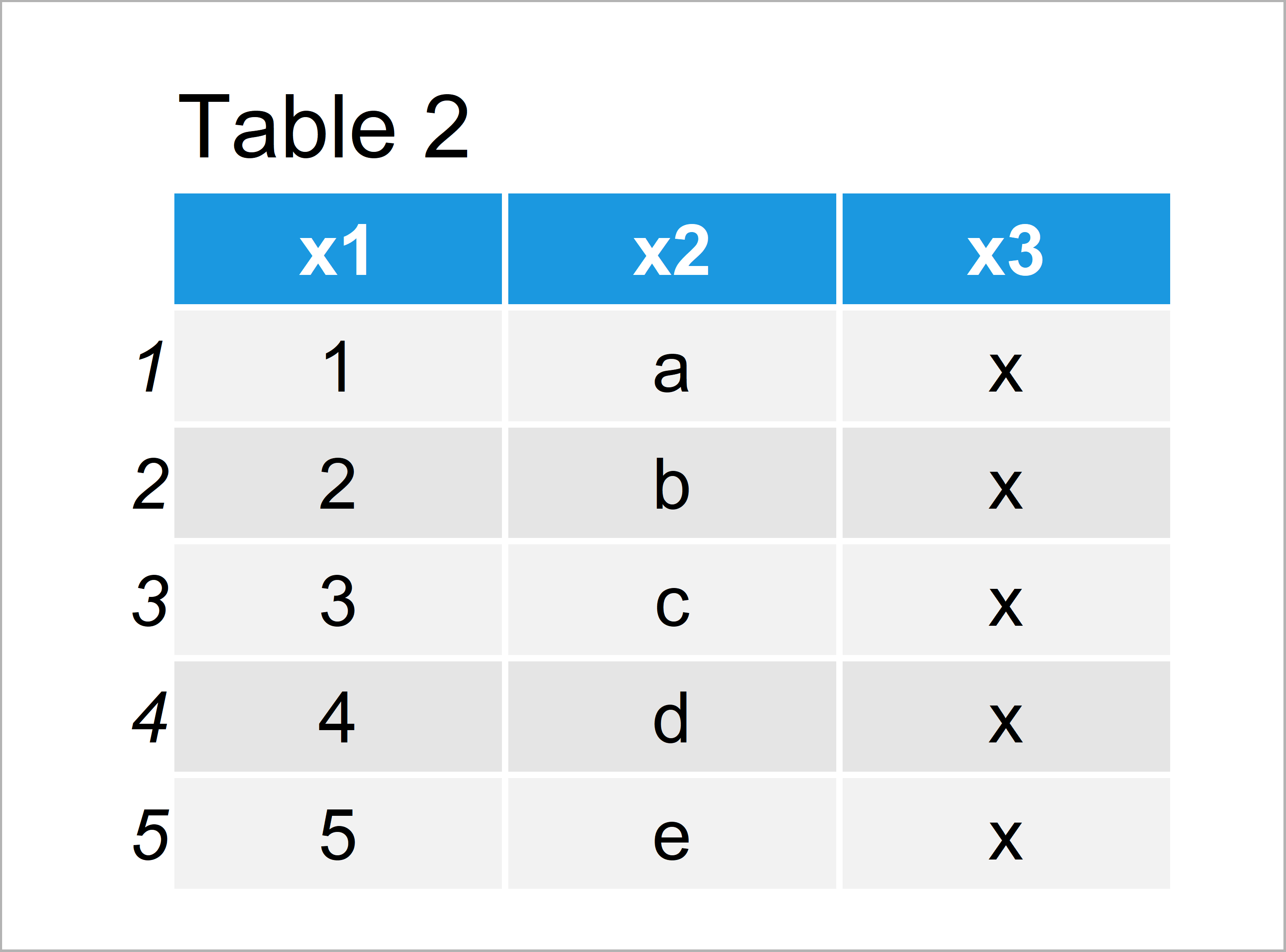# Check If Two Data Frames are the Same in R (2 Examples)

In this tutorial you’ll learn how to test if two data frames contain identical columns in the R programming language.

The tutorial will consist of these topics:

Let’s just jump right in:

## Introduction of Example Data

We use the following three data frames as basement for this R tutorial:

```data1 <- data.frame(x1 = 1:5,    # Create first example data frame
x2 = letters[1:5],
x3 = "x")
data1                            # Print first example data frame```As you can see based on Table 1, our first example data frame contains five rows and three columns x1, x2, and x3.

Let’s create another data frame:

```data2 <- data.frame(x1 = 1:5,    # Create second example data frame
x2 = letters[1:5],
x3 = "x")
data2                            # Print second example data frame```By running the previous syntax we have managed to create Table 2, i.e. another data frame with the variables x1, x2, and x3. You may already have noticed that data1 and data2 are identical, but more on that later.

Let’s create another data frame in R:

```data3 <- data.frame(x1 = 1:5,    # Create third example data frame
x2 = letters[1:5],
x3 = "y")
data3                            # Print third example data frame```The output of the previous code is shown in Table 3: Another data frame containing variables called x1, x2, and x3. Note that the values in the third column are different compared to the previously created data frames.

## Example 1: Check Whether Two Data Frames are Equal Using identical() Function

In this section, I’ll illustrate how to apply the identical function to test if two data frames are equal to each other. Let’s first compare data1 and data2:

```identical(data1, data2)          # Compare first & second data
#  TRUE```

The RStudio console returns the logical value TRUE, i.e. our two data frames data1 and data2 are the same.

Let’s apply the identical function to data1 and data3:

```identical(data1, data3)          # Compare first & third data
#  FALSE```

This time, the RStudio console prints the logical value FALSE, i.e. data1 and data3 are not the same.

## Example 2: Check Whether Two Data Frames are Equal Using all.equal() Function

Alternatively to the identical function, we can also use the all.equal function. As in the previous example, let’s first compare data1 and data2:

```all.equal(data1, data2)          # Compare first & second data
#  TRUE```

The all.equal function also returns the logical value TRUE.

Let’s do the same for data1 and data3:

```all.equal(data1, data3)          # Compare first & third data
#  "Component “x3”: 5 string mismatches"```

Instead of the logical value FALSE, the all.equal function returns a hint where our data frames are different (i.e. in the column x3).

Depending on how you want to use the information whether your data frames are the same, you may prefer the identical function or the all.equal function.

## Video & Further Resources

Do you need further information on the R codes of this tutorial? Then I recommend having a look at the following video of my YouTube channel. In the video, I’m explaining the R programming syntax of this article in a live programming session.

Please accept YouTube cookies to play this video. By accepting you will be accessing content from YouTube, a service provided by an external third party.If you accept this notice, your choice will be saved and the page will refresh.

In addition, you might have a look at the related articles on my website. Some tutorials on topics such as graphics in R, ggplot2, merging, and missing data are shown below.

In this tutorial, I have shown how to compare two data frames and check if they are the same in R programming. Let me know in the comments section, in case you have further questions. In addition, please subscribe to my email newsletter to receive updates on new articles.

Subscribe to the Statistics Globe Newsletter